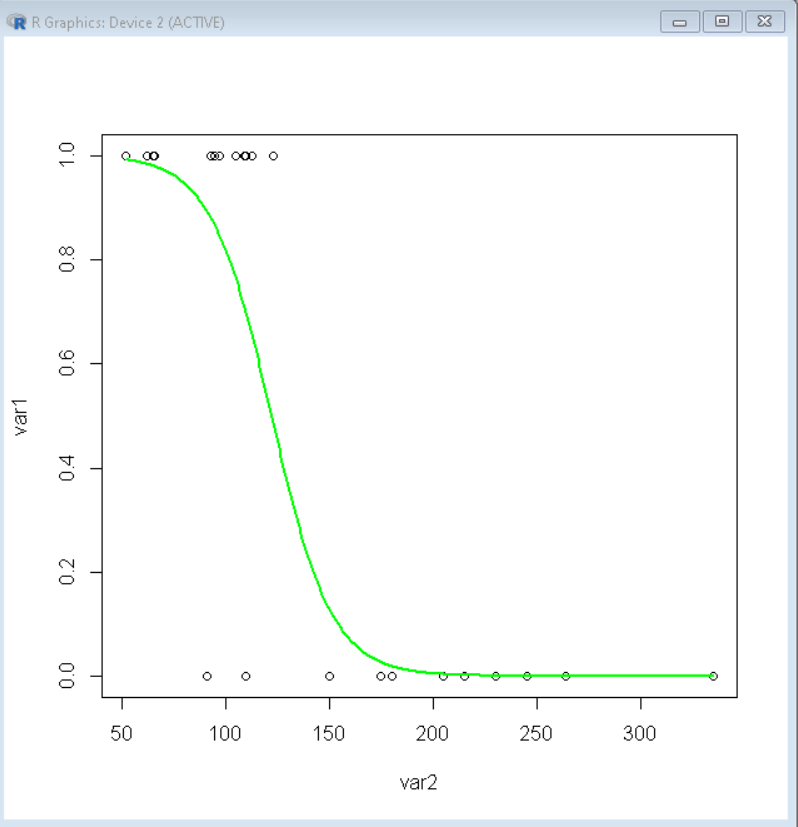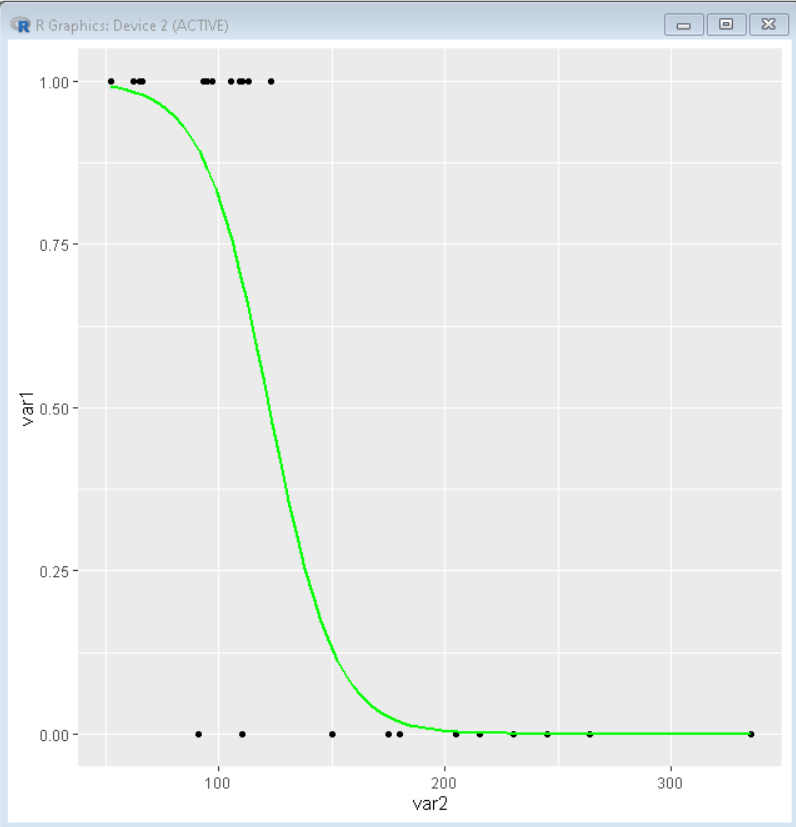# How to Plot a Logistic Regression Curve in R?

• Last Updated : 27 Jan, 2022

In this article, we will learn how to plot a Logistic Regression Curve in the R programming Language.

Logistic regression is basically a supervised classification algorithm. That helps us in creating a differentiating curve that separates two classes of variables. To Plot the Logistic Regression curve in the R Language, we use the following methods.

Dataset used: Sample4

## Method 1:  Using Base R methods

To plot the logistic regression curve in base R, we first fit the variables in a logistic regression model by using the glm() function. The glm() function is used to fit generalized linear models, specified by giving a symbolic description of the linear predictor. Then we use that model to create a data frame where the y-axis variable is changed to its predicted value derived by using the predict() function with the above-created model. Then we plot a scatter plot of original points by using the plot() function and predicted values by using the lines() function.

Syntax:

logistic_model <- glm( formula, family, dataframe )

plot( original_dataframe )

lines( predicted_dataframe )

Parameter:

• formula: determines the symbolic description of the model to be fitted.
• family: determines the description of the error distribution and link function to be used in the model.
• dataframe: determines the data frame to be used for fitting purpose

Example: Plot logistic regression

## R

 `# load dataframe``df <- ``read.csv``(``"Sample4.csv"``)` `# create logistic regression model``logistic_model <- ``glm``(var1 ~ var2, data=df, family=binomial)` `#Data frame with hp in ascending order``Predicted_data <- ``data.frame``(var2=``seq``(``  ``min``(df\$var2), ``max``(df\$var2),len=500))` `# Fill predicted values using regression model``Predicted_data\$var1 = ``predict``(``  ``logistic_model, Predicted_data, type=``"response"``)` `# Plot Predicted data and original data points``plot``(var1 ~ var2, data=df)``lines``(var1 ~ var2, Predicted_data, lwd=2, col=``"green"``)`

Output:## Method 2: Using ggplot2 package

To plot the logistic curve using the ggplot2 package library, we use the stat_smooth() function. The argument method of function with the value “glm” plots the logistic regression curve on top of a ggplot2 plot. So, we first plot the desired scatter plot of original data points and then overlap it with a regression curve using the stat_smooth() function.

Syntax:

plot + stat_smooth( method=”glm”, se, method.args )

Parameter:

• se: determines a boolean that tells whether to display confidence interval around smooth.
• method.args: determines the method function for logistic curve.

Example: Plot logistic regression

## R

 `# load library ggplot2``library``(ggplot2)` `# load data from CSV``df <- ``read.csv``(``"Sample4.csv"``)` `# Plot Predicted data and original data points``ggplot``(df, ``aes``(x=var2, y=var1)) + ``geom_point``() +``      ``stat_smooth``(method=``"glm"``, color=``"green"``, se=``FALSE``,``                ``method.args = ``list``(family=binomial))`

Output:My Personal Notes arrow_drop_up